Author: Oscar Cronquist Article last updated on May 06, 2022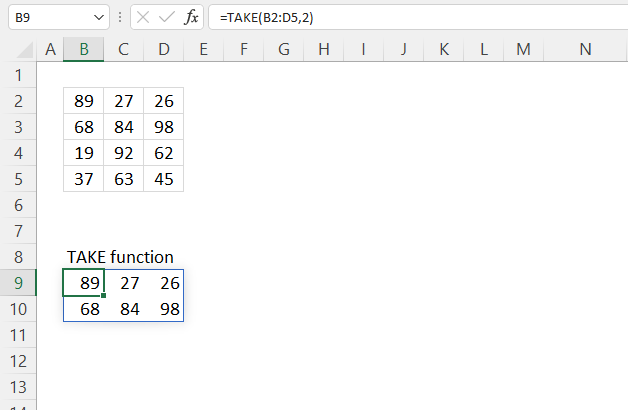The TAKE function returns a given number of rows or columns from a 2D cell range or array.

Dynamic array formula in cell B8:

=TAKE(B2:D5, 2)

The TAKE function is available to Excel 365 users and is in the "Array manipulation" category.

## 1. TAKE Function Syntax

TAKE(array, rows, [columns])

## 2. TAKE Function Arguments

 array Required. The source cell range or array. rows Required. The number of contiguous rows to return, a negative number returns contiguous rows from the end. [columns] Optional. The number of contiguous columns to return, a negative number returns contiguous rows from the end.

## 3. TAKE Function example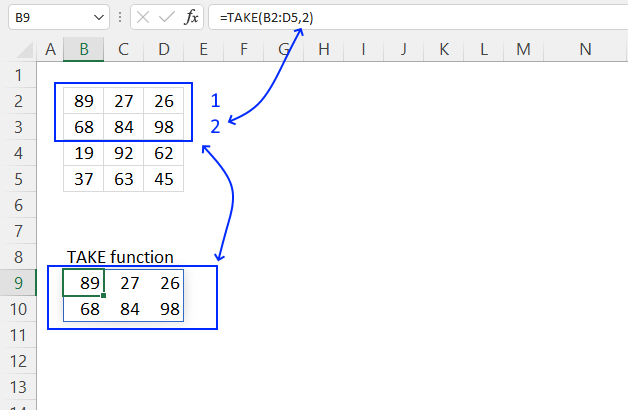The picture above shows how the TAKE function returns the two first rows from cell range B2:D5.

Dynamic array formula in cell B9:

=TAKE(B2:D5, 2)
Note! If any of the values in the source range change, the values in the TAKE function output change as well.

### 3.1 Explaining formula

#### Step 1 - TAKE function

TAKE(array, rows, [columns])

array - B2:D5
rows - 2
[columns] -

#### Step 3 - Evaluate function

TAKE(B2:D5, 2)

becomes

TAKE({89, 27, 26;68, 84, 98;19, 92, 62;37, 63, 45}, 2)

and returns

{89, 27, 26;
68, 84, 98}

## 4. TAKE Function example - return columns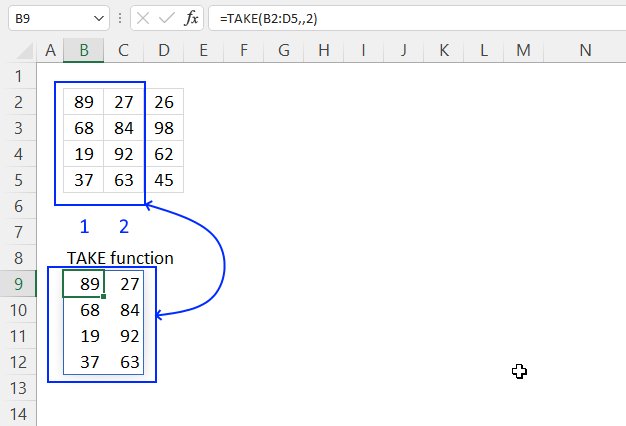The image above demonstrates how to return columns using the TAKE function.

Formula in cell B6:

=TAKE(B2:D5, , 2)

## 5. TAKE Function example - return rows and columns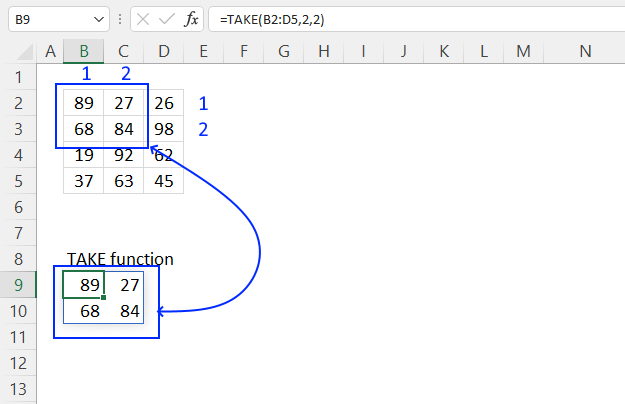The image above demonstrates how to return both rows and columns using the TAKE function. Note that it only returns the intersection of the chosen rows and columns, see the image below.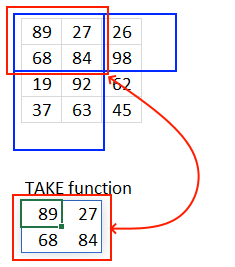Formula in cell B9:

=TAKE(B2:D5, 2, 2)

TAKE(array, rows, [columns])

## 6. TAKE Function example - negative values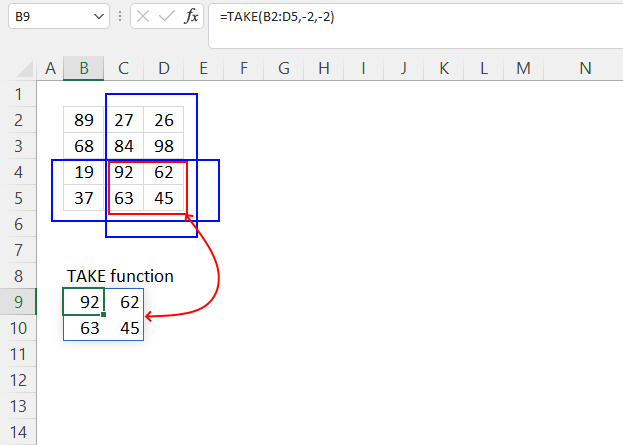The TAKE function lets you use negative arguments, this means that the function returns rows/columns from the end.

Formula in cell B9:

=TAKE(B2:D5, -2, -2)

TAKE(array, rows, [columns])

## 7. TAKE function alternative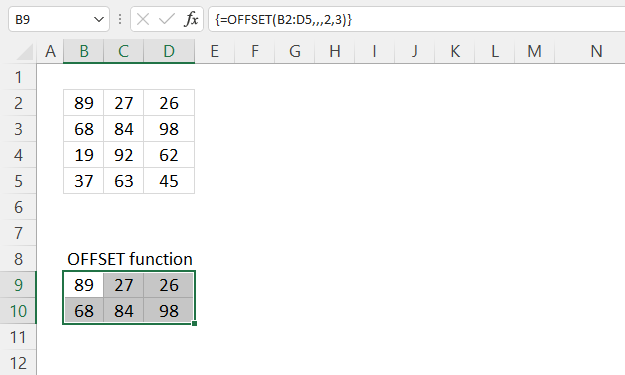It is possible to return the two first rows using the OFFSET function, however, the OFFSET function is volatile and may slow down your worksheet if you use many of them.

Array formula in cell B9:

=OFFSET(B2:D5,,,2,3)

This array formula should work in most Excel versions.

### 7.1 How to enter an array formula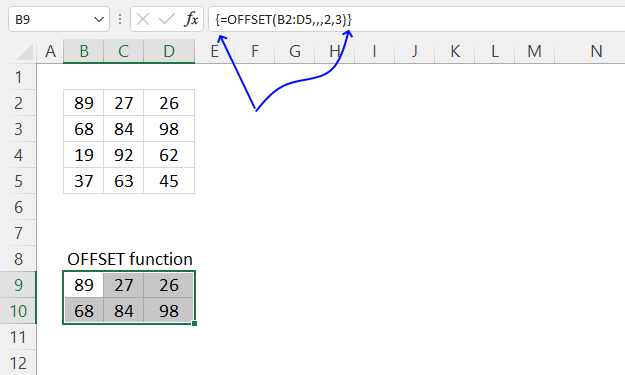1. Double press with left mouse button on with the left mouse button on cell B9, a prompt appears.
2. Type or copy/paste the formula to cell B9.
3. Press and hold CTRL + SHIFT simultaneously.
4. Press Enter once.
5. Release all keys.

The formula now has leading and trailing curly brackets, don't enter these characters yourself. They appear automatically.

### 7.2 Explaining formula in cell B6

#### Step 1 - OFFSET function

The OFFSET function returns a reference to a range that is a given number of rows and columns from a given reference.

OFFSET(reference, rows, columns, [height], [width])

#### Step 2 - Populate arguments

reference - B2:D5
rows -
columns -
[height] - 2
[width] - 3

#### Step 3 - Evaluate OFFSET function

OFFSET(B2:D5,,,2,3)

becomes

OFFSET({89, 27, 26;68, 84, 98;19, 92, 62;37, 63, 45},,,2,3)

and returns

{89, 27, 26; 68, 84, 98}.

## 8. TAKE function - values in random order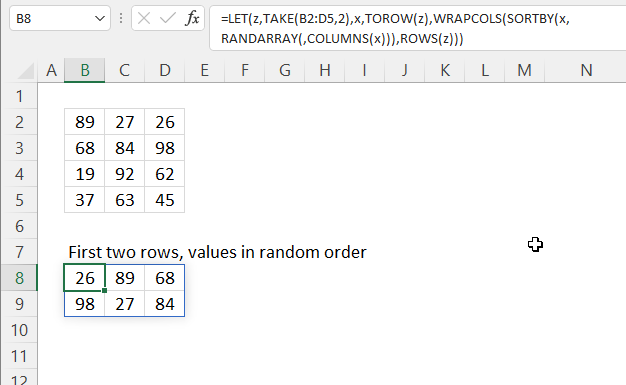The image above shows a formula that returns values in random order from the two first rows in a 2D cell range.

Dynamic array formula in cell B8:

=LET(z,TAKE(B2:D5,2),x,TOROW(z),WRAPCOLS(SORTBY(x,RANDARRAY(,COLUMNS(x))),ROWS(z)))

### 8.1 Explaining formula in cell B8

=WRAPCOLS(SORTBY(TOROW(TAKE(B2:D5,2)),RANDARRAY(,COLUMNS(TOROW(TAKE(B2:D5,2))))),ROWS(TAKE(B2:D5,2)))

#### Step 1 - Extract two first rows

TAKE(B2:D5, 2)

becomes

TAKE({89, 27, 26;68, 84, 98;19, 92, 62;37, 63, 45}, 2)

and returns

{89, 27, 26;68, 84, 98}.

#### Step 2 - Rearrange values to a single row

The TOROW function rearranges values from a 2D cell range or array to a single row.

TOROW(array, [ignore], [scan_by_col])

TOROW(TAKE(B2:D5,2))

becomes

TOROW({89, 27, 26;68, 84, 98})

and returns

{89, 27, 26, 68, 84, 98}.

#### Step 3 - Count cells

The COLUMNS function returns the number of columns in a given cell range or array.

COLUMNS(array)

COLUMNS(TOROW(TAKE(B2:D5,2)))

becomes

COLUMNS({89, 27, 26, 68, 84, 98})

and returns 6.

#### Step 4 - Count rows

The ROWS function returns the number of rows in a given cell range or array.

ROWS (array)

ROWS(TAKE(B2:D5,2))

becomes

ROWS({89, 27, 26; 68, 84, 98})

and returns 2.

#### Step 5 - Create random decimal numbers

The RANDARRAY function returns a table of random numbers across rows and columns.

RANDARRAY([rows], [columns], [min], [max], [whole_number])

RANDARRAY(,COLUMNS(TOROW(TAKE(B2:D5,2))))

becomes

RANDARRAY(,6)

and returns

{0.215398134613085, 0.390607168196479, ... ,0.83231474462401}.

#### Step 6 - Rearrange values in random order

The SORTBY function allows you to sort values from a cell range or array based on a corresponding cell range or array.

SORTBY(array, by_array1, [sort_order1], [by_array2, sort_order2],…)

SORTBY(TOROW(TAKE(B2:D5,2)),RANDARRAY(,COLUMNS(TAKE(B2:D5,2))))

becomes

SORTBY({89, 27, 26, 68, 84, 98}, {0.215398134613085, 0.390607168196479, ... ,0.83231474462401})

and returns

{84, 89, 26, 27, 98, 68}.

#### Step 7 - Rearrange values to original array size

The WRAPCOLS function rearranges values from a single row to a 2D cell range based on a given number of values per column.

WRAPCOLS(SORTBY(TOROW(TAKE(B2:D5,2)),RANDARRAY(,COLUMNS(TOROW(TAKE(B2:D5,2))))),ROWS(TAKE(B2:D5,2)))

becomes

WRAPCOLS({84, 89, 26, 27, 98, 68},ROWS(TAKE(B2:D5,2)))

becomes

WRAPCOLS({84, 89, 26, 27, 98, 68}, 2)

and returns

{84, 89, 26; 27, 98, 68}

#### Step 8 - Shorten formula

The LET function lets you name intermediate calculation results which can shorten formulas considerably and improve performance.

LET(name1name_value1calculation_or_name2, [name_value2calculation_or_name3...])

WRAPCOLS(SORTBY(TOROW(TAKE(B2:D5,2)),RANDARRAY(,COLUMNS(TOROW(TAKE(B2:D5,2))))),TOROW(TAKE(B2:D5,2)))

becomes

LET(z,TAKE(B2:D5,2),x,TOROW(z),WRAPCOLS(SORTBY(x,RANDARRAY(,COLUMNS(x))),ROWS(z)))

## 9. TAKE Function - multiple source ranges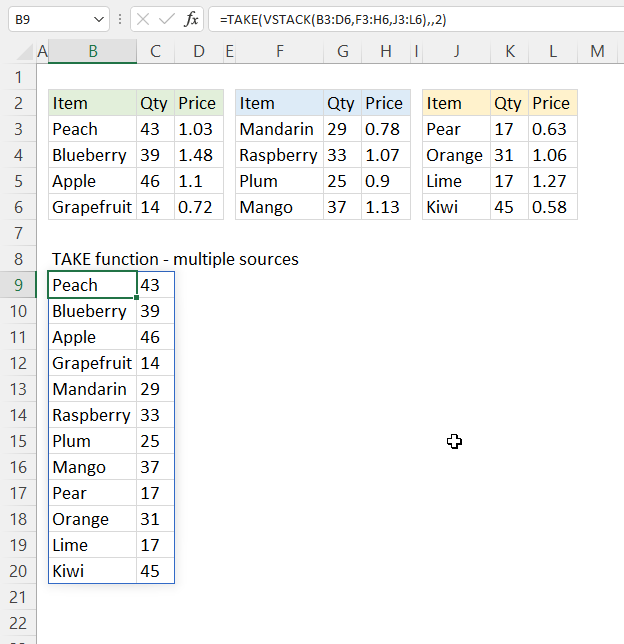The picture above shows a formula that merges three non-contiguous cell ranges and return values from the two first columns.

Dynamic array formula in cell B9:

=TAKE(VSTACK(B3:D6, F3:H6, J3:L6), , 2)

### 9.1 Explaining formula

#### Step 1 - Stack values horizontally

The VSTACK function lets you combine cell ranges or arrays, it joins data to the first blank cell at the bottom of a cell range or array (vertical stacking)

VSTACK(array1, [array2],...)

VSTACK(B3:D6, F3:H6, J3:L6)

becomes

VSTACK({"Peach", 43, 1.03;"Blueberry", 39, 1.48;"Apple", 46, 1.1;"Grapefruit", 14, 0.72}, {"Mandarin", 29, 0.78;"Raspberry", 33, 1.07;"Plum", 25, 0.9;"Mango", 37, 1.13}, {"Pear", 17, 0.63;"Orange", 31, 1.06;"Lime", 17, 1.27;"Kiwi", 45, 0.58})

and returns

{"Peach", 43, 1.03;
"Blueberry", 39, 1.48;
"Apple", 46, 1.1;
"Grapefruit", 14, 0.72;
"Mandarin", 29, 0.78;
"Raspberry", 33, 1.07;
"Plum", 25, 0.9;
"Mango", 37, 1.13;
"Pear", 17, 0.63;
"Orange", 31, 1.06;
"Lime", 17, 1.27;
"Kiwi", 45, 0.58}

#### Step 2 - Wrap values to rows

TAKE(VSTACK(B3:D6, F3:H6, J3:L6), , 2)

becomes

TAKE({"Peach", 43, 1.03;
"Blueberry", 39, 1.48;
"Apple", 46, 1.1;
"Grapefruit", 14, 0.72;
"Mandarin", 29, 0.78;
"Raspberry", 33, 1.07;
"Plum", 25, 0.9;
"Mango", 37, 1.13;
"Pear", 17, 0.63;
"Orange", 31, 1.06;
"Lime", 17, 1.27;
"Kiwi", 45, 0.58}, , 2)

and returns

{"Peach", 43;
"Blueberry", 39;
"Apple", 46;
"Grapefruit", 14;
"Mandarin", 29;
"Raspberry", 33;
"Plum", 25;
"Mango", 37;
"Pear", 17;
"Orange", 31;
"Lime", 17;
"Kiwi", 45}